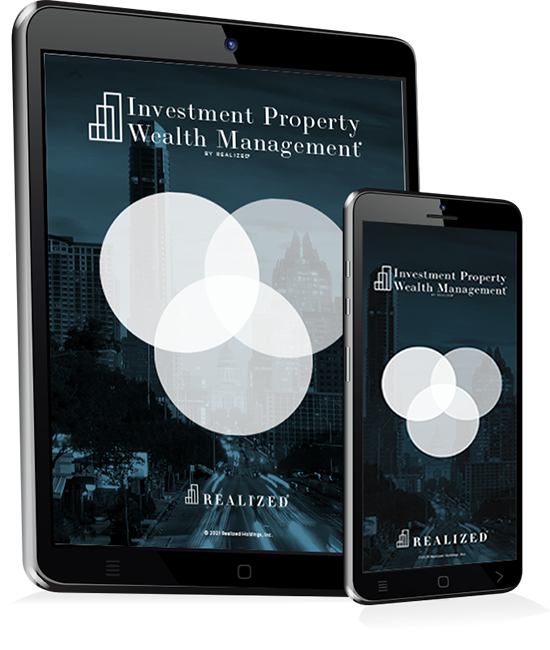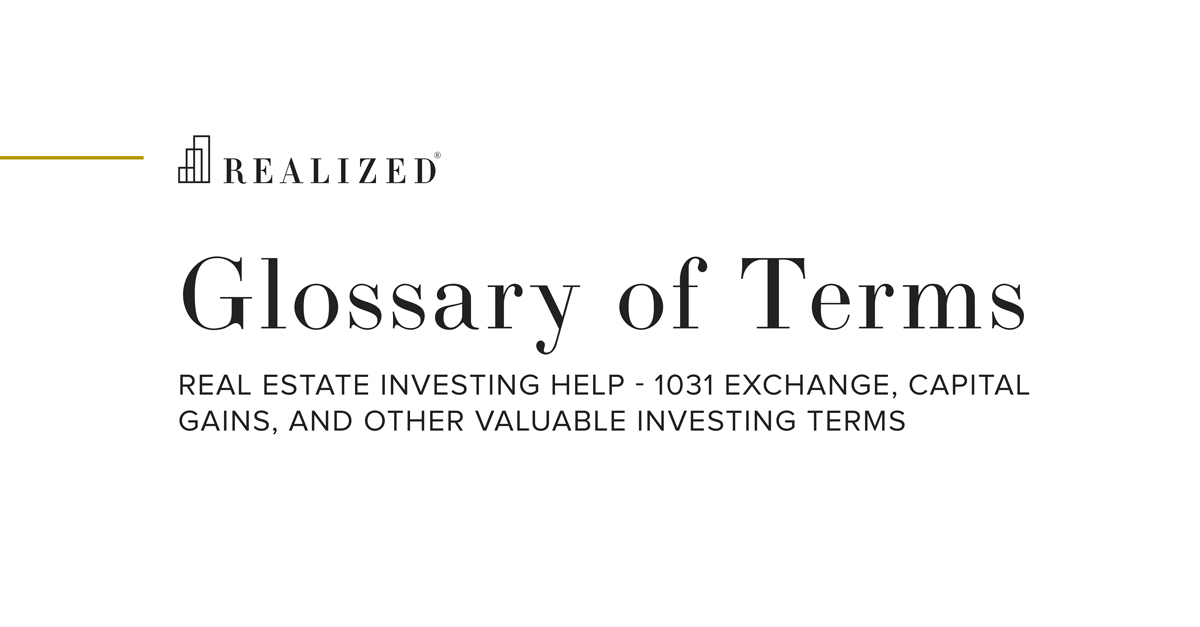Terminal Value 2021-05-07 08:00:00

The value of an investment at the end of its holding period. In the context of commercial real estate, the terminal value of an investment property is often estimated by applying a terminal cap rate to its projected net operating income (NOI) at the time of sale, or the year following sale.

For example, if an investment property has an anticipated holding period of 7 years, an investor may project the NOI of the property in year 8 to be \$130,000. If the investor applies a 7.0% terminal cap rate, then the estimated terminal value would be \$1,857,715 (NOI of \$130,000 divided by terminal cap rate of 7.0%). Also known as the exit value.

An income-producing property can theoretically continue producing income indefinitely. However, most investors want a return of principal at some point. That is where the terminal value comes in. It’s basically a bookend to close out the investment.

How does an investor set the exit cap rate? For a business, it may use the liquidation value as part of its terminal value. For income-producing real estate property, which will continue for the buyer, assets will obviously not be liquidated. The investor will need to use a different method for figuring out the exit cap rate.

In general, the seller can assume a cap rate at the terminal value that a buyer will use. This exit cap rate might be calculated by looking at the first-year cap rate (i.e., 6.3%) and then the seventh-year cap rate of 7%. That equals an approximately 0.1% annual rate of growth.

But what if the investor is only in year five and wants to determine an exit cap rate for year ten? It’s the same formula. The investment starts year one with a 6.3% cap rate. Then in year five, it has risen to 6.7%. With a 0.1% annual growth rate, the investor can project that year ten will have a 7.3% cap rate. Based on experience, the investor may have some other method for determining the exit cap rate. Additionally, historical cap rate data can be purchased (but may be expensive).